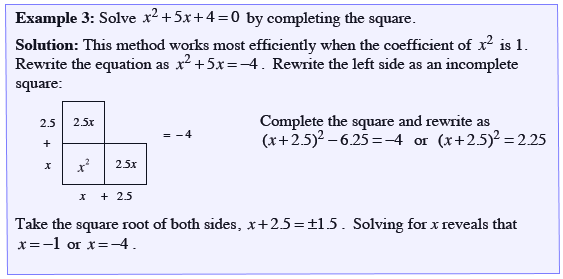Home > CCA > Chapter 9 > Lesson 9.3.1 > Problem9-76

9-76.

For the quadratic function $f(x)=x^2+6x+11$: Homework Help ✎

1. Use the idea of completing the square to write it in graphing form.

Use the Math Notes box.2. State the vertex and sketch a graph of the parabola.

In the standard equation, the vertex is ($h,k$).

$(−3,2)$

3. Use the graph to explain why the equation $x^2+6x+11=0$ has no real solutions.

Are there any $x$-intercepts?